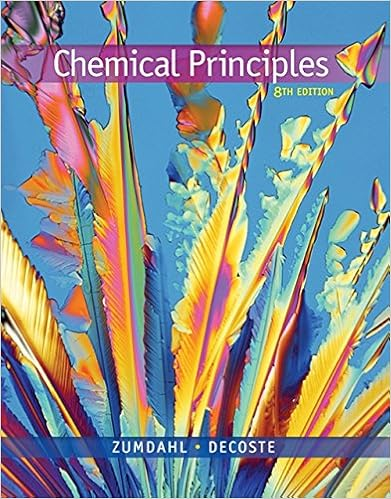# CHMY_171_practice_exam_4.doc - CHMY 171 PRACTICE EXAM IV...

• Test Prep
• coolmanbam258
• 5

This preview shows page 1 - 3 out of 5 pages.

##### We have textbook solutions for you!
The document you are viewing contains questions related to this textbook.The document you are viewing contains questions related to this textbook.
Chapter 13 / Exercise 43
Chemical Principles
DeCoste/ZumdahlExpert Verified
CHMY 171 PRACTICE EXAM IV Watch your significant figures and units throughout the exam! NAME _____________________ 1. Titanium a strong lightweight corrosion-resistant metal is prepared by the reduction of titanium(IV)chloride with molten magnesium: TiCl 4 + 2Mg Ti + 2MgCl Part. A. (6 pt) Calculate the theoretical yield of Ti if 148kg of TiCl 4 reacts with 46.5 kg of Mg. Part B. (6 pt) Calculate the % yield if 24345 g of Ti was isolated from the reaction. Part C. (4 pt) If a percent yield of greater than 100% had been observed, what errors were likely made? 2. (6 pt) What is the mass percent oxygen in Na 2 CO 3 3. (3 pt) Label the following solutions as strongly basic, weakly basic, strongly acidic, or weakly acidic. 2 ? b) 0.120 M NaOH c) 0.150 M HI d) 0.040 M NH 3
##### We have textbook solutions for you!
The document you are viewing contains questions related to this textbook.The document you are viewing contains questions related to this textbook.
Chapter 13 / Exercise 43
Chemical Principles
DeCoste/ZumdahlExpert Verified
4. (4 pt) Complete and balance the following neutralization reaction. HNO 3 + Sr(OH) 2 5. (8 pt) Indicate the oxidation number of the underlined atom in the following compounds. a) Na H b) c) C H 4 O d) 6. (4 pt) Draw an arrow to indicate which direction the following reactions are most likely to proceed. NaH H 2
•••